#The "Quartic Formula"#### Introduction.

Shortly after the discovery of a method to solve the cubic equation, Lodovico Ferrari (1522-1565), a student of Cardano, found a way to solve the quartic equation. His solution is a testimony to both the power and the limitations of elementary algebra.

Our objective is to find two roots of the quartic equation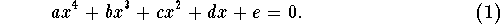The other two roots (real or complex) can then be found by polynomial division and the quadratic formula. The solution proceeds in two steps. First, the quartic equation is "depressed"; then one reduces the problem to solving a related cubic equation.

#### Depressing the quartic equation.

The trick we used to depress the cubic equation, works basically the same way for the quartic equation.

We apply the substitution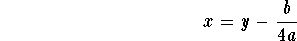to the quartic equation (1) to obtain: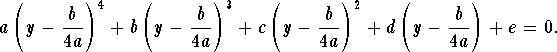Multiplying out and simplifying, we obtain the "depressed" quartic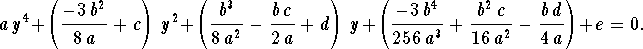Let's try this for the example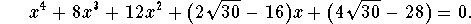Our substitution will be x=y-2; expanding and simplifying, we obtain the depressed quartic equation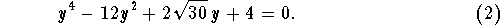#### Solving the depressed quartic.

We are left with solving a depressed quartic equation of the form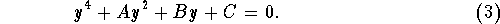We will first move the y-term to the other side.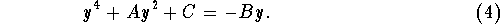Next, we "complete the square" on the left side, but we want to end up with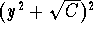. To accomplish that, we add the term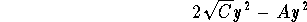on both sides: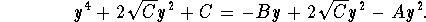Simplifying the left side results in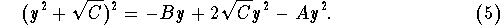Now here comes the crucial trick: Using a new unknown z, we want to change the left side of (5) to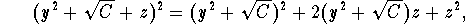which means we have to add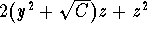to both sides of (5) to obtain: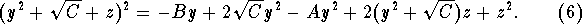What a mess! Let's see what we get for our concrete example Equation (2):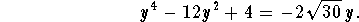"Completing the square" in the fashion indicated above yields: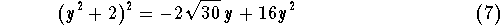Since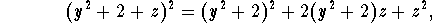adding the term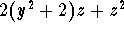to both sides of (7) results in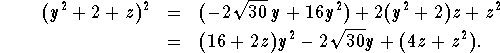The left side is a "perfect square". The next step will be to determine z so that the right side looks like a perfect square as well.

Note that the right side is a quadratic polynomial in y. Ending up with a perfect square is just another way of saying that this polynomial has a repeated factor. A quadratic polynomial has a repeated factor if its discriminant is 0. Let's compute the discriminant in our example: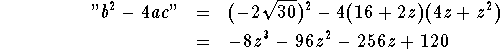This polynomial is called the resolvent cubic polynomial for the quartic equation.

We can find a real root of the resolvent cubic; in this case, the Rational Zero Test reveals that z=-5 is such a root.

Where are we? If z=-5, then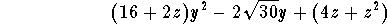is supposed to be a perfect square. Let's check that.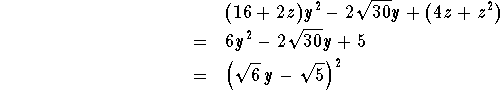Bingo! By now, Equation (7) looks like this: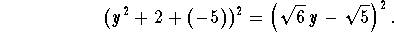We're nearly done. Let's take square roots on both sides: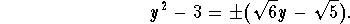We discard one of the two choices and end up with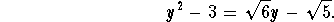This is a quadratic equation! Its roots are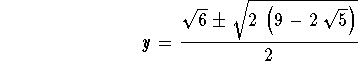Using the substitution x=y-2, we see that our original equation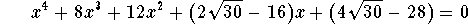has two roots of the form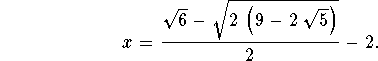The other two roots can be found by polynomial long division, and application of the quadratic formula.

Let me finish by convincing you that making the right side a perfect square always amounts to solving a cubic equation: In the last general step (Equation (6)), we had written our depressed quartic equation as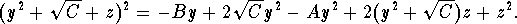Writing the right side neatly as a polynomial in y, we obtain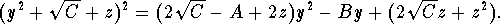The discriminant of the right side is therefore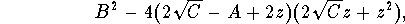a cubic polynomial in z.

Thus the procedure outlined will work in general. One word of caution: A quartic equation may have four complex roots; so you should expect complex numbers to play a much bigger role in general than in my concrete example. In my discussion of the general case, I have, for example, tacitly assumed that C is positive.

For an interesting account of Euler's approach to solving quartic equations, see the article The quartic equation: invariants and Euler’s solution revealed by RWD Nickalls.

#### Exercise 1.

Find the resolvent cubic polynomial for the depressed quartic equation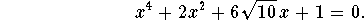Check that z=3 is a root of the resolvent cubic for the equation, then find all roots of the quartic equation.

#### Exercise 2.

Explain the relationship between the method of "completing the square" and the method of "depressing" a cubic or quartic polynomial.[Back]
[Algebra] [Trigonometry] [Complex Variables]
[Calculus] [Differential Equations] [Matrix Algebra]S.O.S MATHematics home page

Do you need more help? Please post your question on our S.O.S. Mathematics CyberBoard.Helmut Knaust
Mon Jun 30 13:10:51 MDT 1997